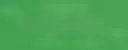# Data Structures And Algorithms: Arrays In PythonAn array is a collection of similar, sequential data types stored in a central location.

Introduction

By a collection of similar data types I mean if we want to store whole numbers/integers we can have our array that only contains integer elements, the same applies for strings, floats, and other data types.

Let’s say we have x = 100, this means we have declared variable x with a value of 100 in memory.

Memory is made up of bytes, one byte is 8 bits so we can describe memory as a long tape of bytes.

When we run x = 100, the memory manager allocates 4 bytes in memory, integer elements are usually allocated 4 bytes.

Remember 1 byte is 8 bits, so 4 bytes is 4*8 which is 32 bits, our integer 100 is converted into binary (10101110) as 29 zeros and then 101.

00000000

0000000)

00000000

00000101

The binary number is stored in memory, the 4 bytes are allocated 4 spaces in memory with 8 bits to each section.

Now if we wanted to store 10 student values, we would need 10 variables:

We can see this is a tedious and repetitive task, hence the need for arrays arose.

Creating An Array

In Python we have to import the array module before we can create an array, we have three methods to choose from:

• Importing array using it’s original name – array.

• Importing array using it’s alias name – array.

• Importing all functions using the asterisks (*)

When we use the asterisk * it means we want to import all the functions.

Let me explain the above code line by line, first line we import our array module, using the asterisks method.

Second in we create our array, the name of our array is vals meaning values. Then we assign an array to vals and within the parenthesis, with “i" we include the type of array and set of integers for the size of the array.

The array type is specified using the type code table below.

We have chosen normal integer, hence we are using the symbol i from the type code table, small i indicates we can have positive and negative integers in our array.

Then we have our square brackets[] to indicate an array then fill in the elements.

Line three, we utilize the print function to display elements in our array named vals.

Printing Individual Elements In An Array

Now that we have our array, time to play with it, lets print individual values from the array.

To do that we will use index numbers.

Array Operations

In arrays we can use certain functions when we want to add , remove, append elements in the array or if we simply want to check the type of the array.

The append() function:

Takes in a single item. In this case an integer 22 is to be added and is automatically sent to the end of the list.

The remove() function:

Removes a specific element in the array. Within the code below we have removed 45.

The pop() function:

This method removes the value at the end of the array.

The reverse() function:

The name us quite explanatory, it reverses items in the array.

The len function:

Used when we do not know the length

Array Traversal:

We have reversed, deleted, appended elements in our array. Now what if we want to print all the elements in the array and not just a single element.

Well we can use a loop to traverse through the array, it will start from index 0 print the element and jump to the next index.

Join other developers and claim your FAUN account now!### The Maths Geek 🤓

@thenjikubheka
Mathematician | Software Engineer | Amazonian| Open Source | Blogger | Ban Killer Robots

Influence

Total Hits

Posts